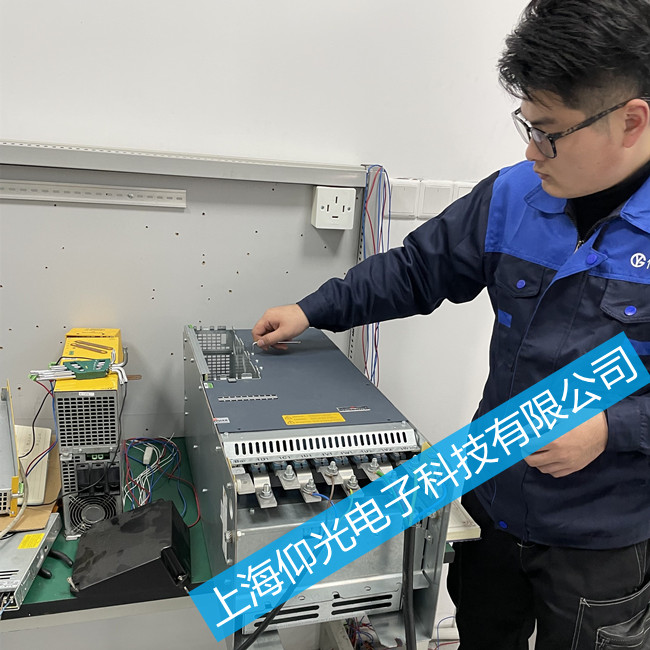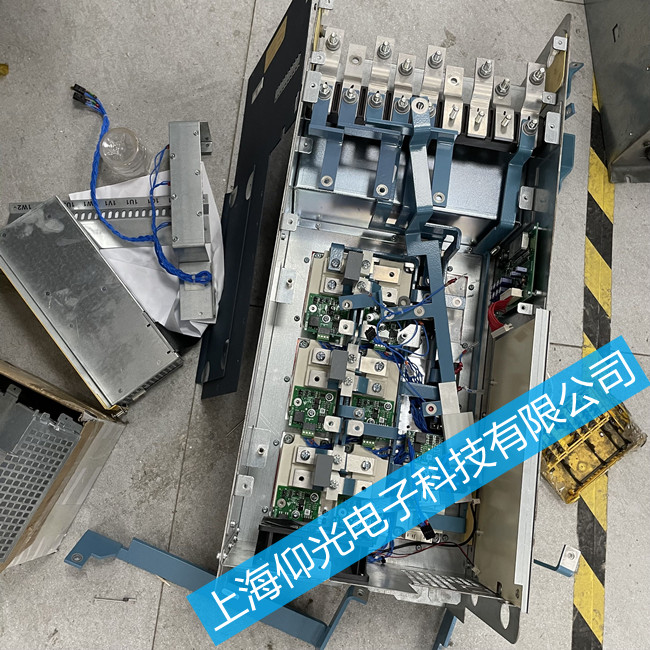Hi，欢迎访问上海仰光电子科技有限公司！

包米勒伺服驱动器维修BM4454-FI1-01243-03报警维修苏州，有无显示，缺相，过流，过压，欠压，过热，过载，接地，参数错误， 有显示无输出，模块损坏，报错。显示1 显示2 数码管报警F001,F002等，百格拉驱动器，施耐德变频器，上海维修变频器，浙江维修驱动器，苏州维修变频器，温州维修变频器，北京维修变频器32 =超时协议
33 =协议结构
34 =错误的模块类型
35 =电报中的数据太多
36 =电报中的数据不足
37 =操作数无效
38 =无效的内存类型
39 =无效的操作数地址
41=值大于最大值
42 =参数被写保护
43 =此操作状态下的参数不可写
44 =参数值无效
45 =通信错误WinBASS控制器
46-47 =保留

48 =功能模块A中的错误
49 =功能模块B出错
50 =功能模块C中的错误
51 =功能模块D中的错误
52 =功能模块E中的错误
53 =选件模块G出错
54 =选件模块H出错
55 =选件模块J中的错误
56 =选件模块K出错
57 =选件模块L出错
58 =选项模块M中的错误
59 =等待从站的RST信号时超时
60 = SPI传输模块f控制器中的CRC错误
61 = SPI传输控制器模块中的CRC错误
62-63 =保留

64 =主电源故障
65 =相故障
66 =主电源欠压
67 =主电源过压
68 =欠压24V
69-78 =保留
79 = 保留

80 = HIPER-FACE规范后的通信错误
81 =散热片温度
82 = U DC链路过压
83=过电流
84 =接地电流
85 =设备内部温度过高
86 =断线温度传感器
87 =安全继电器关闭（或缺陷）
88 =桥接器短路
89 =功率单元未准备好运行
90 =阶段失败
91电源故障恢复主电源
92 =主电源欠压
93 =主电源过压
94 =欠压U DC链路
95 = 保留

【 我们确保修好测试好给客户！！！！】